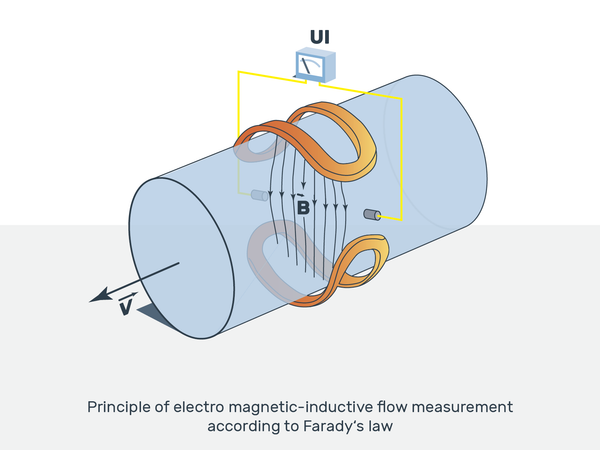# Electromagnetic Flowmeter

Filter

Electromagnetic Flow Meters are based on FARADAY'S LAW OF INDUCTION. These meters are also called as Magflow or Electromagnetic Flowmeters. A magnetic field is applied to the metering tube, which results in a potential difference proportional to the flow velocity perpendicular to the flux lines. The physical principle at work is electromagnetic induction and mathematically defined as E=k*B*D*V.The induced voltage (E) is directly proportional to the velocity (V) of the fluid moving through the magnetic field (B). The induced voltage is carried to the transmitter through the electrode circuit. The transmitter then converts this voltage into a quantifiable flow velocity. The volumetric flow rate of the fluid is calculated using this known velocity along with the area of the pipe.

When a flowmeter is installed and activated, its operations begin with a pair of charged magnetic coils. As energy passes through the coils, they produce a magnetic field that remains perpendicular to both the conductive fluid being measured and the axis of the electrodes taking measurements. The fluid moves along the longitudinal axis of the flowmeter, making any generated induced voltage perpendicular to the field and the fluid velocity. An increase in the flow rate of the conductive fluid will create a proportionate increase the voltage level.

17 products

17 products